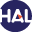# Volume 3 N°1 (2006)

Latest addition : 29 December 2010.

• ### Computing two dimensional flood wave propagation

6 December 2006,

Full Title: Computing two dimensional flood wave propagation using unstructured finite volume method: Application to the Ourika valley

Abstract:

This study is devoted to the flood wave propagation modellingfl corresponding to a realistic situation. The equations that governs the propagation of a flood wave, in natural rivers, corresponds to the free surface flow equations in the Shallow Water case. The obtained two dimensional system, known as Saint Venant’s system, is derived from the three-dimensional incompressible Navier Stokes equations by depth-averaging of the state variables. This system is written in a conservative form with hyperbolic homogeneous part. The discretization of the convection part is carried out by the use of the finite volume method on unstructured mesh.

To increase the accuracy of the scheme, the MUSCL technique is used. The diffusive part is discretized using a Green-Gauss interpolation technique based on a diamond shaped co-volume.

For the numerical experiment, we have studied a realistic channel of the Ourika valley which is located in Morocco. The flood occurred on August 1995 is simulated with the objective of evaluating the behavior of the wave propagation in the channel.

The results of the proposed numerical model gives velocities and free surface elevations at diffrent stopped times of the simulation.

Paper presented by Prof. F. BenkhaldounVoir sur HAL : https://hal.archives-ouvertes.fr/hal-01128337

• ### Moving Meshes and Higher Order Finite Volume Reconstructions

6 December 2006,

Full Title: Moving Meshes and Higher Order Finite Volume Reconstructions

Abstract:

In this paper, we present and evaluate a moving mesh Finite Volume method for hyperbolic conservation laws. The method consists of two parts; a mesh moving scheme based on the algorithm of Tang and Tang, and a third order accurate bi-hyperbolic reconstruction which is an extension of Marquina’s PHM. The resulting algorithm calculates the solution of the conservation laws directly in physical space, without any transformation of the computational grid or the hyperbolic equations. Numerical experiments in one and two space dimensions indicate high numerical accuracy of the method.

Publication date: February, 7, 2006Voir sur HAL : https://hal.archives-ouvertes.fr/hal-01121364

• ### Convergence and Sensitivity Analysis of Repair Algorithms in 1D

6 December 2006,

Full Title: Convergence and Sensitivity Analysis of Repair Algorithms in 1D

Abstract:

We prove the convergence of some repair algorithms for linear advection in dimension one. The convergence depends on the size of the box where the distribution of the mass excess is performed. Various numerical examples illustrate the theoretical results. Applications to gas dynamics in dimension one is also discussed.

Paper presented by Prof. R. Abgrall

Publication date: January, 19, 2006Voir sur HAL : https://hal.archives-ouvertes.fr/hal-01114186

• ### FV schemes for a non linear Hyperbolic CL with a flux function involving discontinuous coefficients

6 December 2006,

Full Title: Finite volume schemes for a non linear hyperbolic conservation law with a flux function involving discontinuous coefficients

Abstract:

A model for two phase flow in porous media with distinct permeabilities leads to a non linear hyperbolic conservation law with a discontinuous flux function. In this paper for such a problem, the notion of entropy solution is presented and existence and convergence of a finite volume scheme are proved. No hypothesis of convexity or genuine non linearity on the flux function is assumed, which is a new point in comparison with preceding works. As the trace of the solution along the line of discontinuity of the flux function can not be considered, this problem is more complex. To illustrate these results, some numerical tests are presented.

Paper presented by Prof. F. BenkhaldounVoir sur HAL : https://hal.archives-ouvertes.fr/hal-01114200

• ### A Simple Finite-Volume Method for Compressible Isothermal Two-Phase Flows Simulation

6 December 2006,

Full Title: A Simple Finite-Volume Method for Compressible Isothermal Two-Phase Flows Simulation

Abstract:

We present a simple method for simulating isothermal compressible two-phase flows with mass transfer. The convective part of the model is compatible with the Least Action Principle and the system is endowed with an entropy inequality which accounts for phase change terms and phasic pressure unbalance. A study of the system as a relaxed model of two equilibrium models is performed.

This study allows the design of two-step relaxation-convection Finite-Volume discretization scheme which complies with the entropy balance of the model which drives the mass transfer phase-change process. Numerical results involving dynamical phase-change are presented.

Paper presented by Prof. J.M. HerardVoir sur HAL : https://hal.archives-ouvertes.fr/hal-01114190